1. Please check and comment entries here.

Topic review

Extended Characteristic Polynomial

Subjects: Others
View times: 492

Definition

In the context of molecular topology it is well known the Characteristic Polynomial (ChP) for its important uses in relating the structure with molecular properties of hydrocarbons, as well as a high resolution discriminant for chemical structures. Also it is well known that, as any other topological based descriptor/function, the ChP is blind to the nature of the chemical element and of the chemical bond, both being treated as indistinguishable in the context of the chemical graphs theory. An extension of the Characteristic Polynomial (EChP) is proposed by relaxing the identity matrix and adjacency matrix to contain non-binary based values, by in the same time keeping the meaning of those matrices (identity as collecting information regarding the identity of the atoms in the molecule and adjacency as collecting information regarding the connectivity or bonds in the molecule) and their feature (of being symmetrical).

1. Extending the Characteristic Polynomial

The characteristic polynomial was firstly encountered (at that time so called 'secular equation') in conjunction with the movement of the planets by famous mathematicians: Euler (1743), D’Alembert (1750), Lagrange (1773), Laplace (1775), its theory and use was gradually refined by others: Fourier (1822), Cauchy (1829), Sylvester (1852), Hermite (1857), Weierstrass (1867), Jordan (1872), and Kronecker (Kronecker, 1890).

Once the more general problem were identified as standing for any Hessian matrix by Sylvester (1880) the characteristic polynomial found its use in the approximate treatment of the Schrödinger's equation (1926) for the wavefunction (Hartree, 1928) and Fock (Fock, 1930) finding the same eigenvector-eigenvalue problem as in the Slater's treatment (1929), revised later by Hartree & Hartree (1935).

While the first reports relating to the use of the characteristic polynomial in relation with the chemical structure appears shortly after the discovery of wave-based treatment of microscopic level from Hückel (1931), the roots of this extended version of the characteristic polynomial can be attributed to the assignment of the individual electronic energies (εi), as accounted for the first time by Coulson in 1937, 1940, and 1950.

The characteristic polynomial (ChP) is the natural construction of a polynomial in which the eigenvalues of the [Ad] are the roots of the ChP as it follows:

λ is an eigenvalue of [Ad] ↔ it exists [v] ≠ 0 eigenvector such that λ·[v] = [Ad]·[v] →

(λ·[Id]-[Ad])·[v] = 0; since v ≠ 0 → [λ·Id - Ad] is singular → det([λ·Id - Ad]) = 0

The characteristic polynomial ChP is a polynomial in λ of degree the number of atoms, ChP(λ) = det([λ·Id - Ad]).

As introduced in (Joița & Jäntschi, 2017), the Extended Characteristic Polynomial (EChP) of a chemical structure is defined similarly (see Table 1) with the Characteristic Polynomial (ChP) of its associated topology (T).

Table 1. Topology, Chemistry and Geometry for Ethanimine (HN=CH-CH3)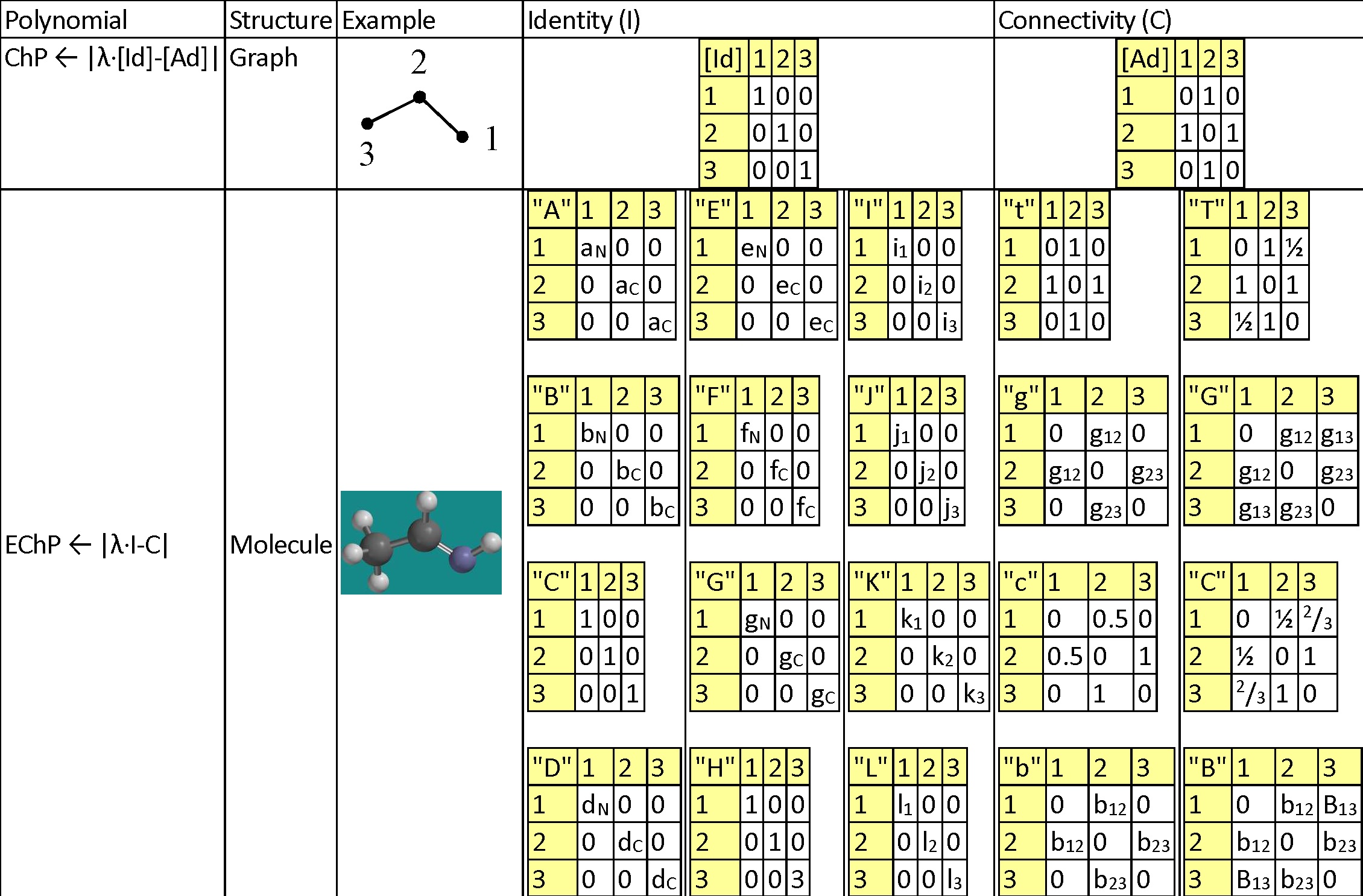Legend for replacement values: aC = 12/294; aN = 14/294; bC = 3915/3915; bN = 77.355/3915; dC = 2267/30000; dN = 1495/30000; eC = 2.55/4.00; eN = 3.04/4.00; fC = 1086.2/1312.0; fN = 1402/1312; gC = 3915/3915; gN = 63.15/3915; i1 = -0.738; i2 = 0.497; i3 = -0.756; j1 = -0.593; j2 = 0.090; j3 = -0.653; k1 = -0.628; k2 = 0.097; k3 = -0.734; l1 = 0.0; l2 = 0.0; l3 = 0.0; g12 = 1/1.290; g13 = 1/2.451; g23 = 1/1.496; b12 = 1/1.869; b23 = 1/0.905; B13 = 1/(1/1.869+1/0.905).

2. Extended Characteristic Polynomial

A natural extension of ChP is to store in the identity matrix (instead of unity) non-unity values accounting for different atoms (and then the [Id] matrix is replaced by a generic matrix, [Ip], having more than one alternative to be filled with values), as well as to store in the adjacency matrix (instead of unity) non-unity values accounting for different bonds (and then the [Ad] matrix is replaced by a generic matrix, [Cq], having more than one alternative to be filled with values). Then the extended characteristic polynomial is defined by EChP(λ; p, q) = det([λ·Ip - Cq]).

The proposed alternatives for identifying the atoms are p ∈ {"A", "B", "C", "D", "E", "F", "G", "H" "I", "J", "K", "L"}, being exemplified in Table 1 and having the following meanings: Atomic mass ("A"), Boiling point ("B"), Count ("C"), Density ("D"), Electronegativity ("E"), First ionization potential ("F"), Melting point ("G"), Hydrogen connections ("H"), Electrostatic charge ("I"), Mulliken charge ("J"), Natural charge ("K"), and Spin ("L").

The proposed alternatives for identifying the bonds are q ∈ {"t", "g", "c", "b", "T", "G", "C", "B"}, being exemplified in Table 1 and having the following meanings: being derived of adjacencies ({"t", "g", "c", "b"}) and on distances {"T", "G", "C", "B"}, as classical topological measures ("t" and "T"), inverses of geometrical distances ("g" and "G"), inverses of conventional bond orders ("c" and "C"), and inverses of Mulliken bond orders ("b" and "B").

It should be noted that all proposed alternatives keep the symmetry alive in both identity (Ip) and connectivity (Cq) replacements of identity matrix ([Id]) and adjacency matrix ([Ad]), keeping thus the Hessian property of their linear combination.

Also it should be noted that any value greater than 1 as entry in any of identity (Ip) and connectivity (Cq) matrices is dangerous to the computations of the associated characteristic polynomial (any number greater than 1 easily diverges producing huge numbers when is risen at a natural power), and as such, proportion (from 0 to 1, or from -1 to 1) scales must be used whenever is possible to keep at reasonably low level the evaluations for the extended version of the characteristic polynomial.

A series of constant values has been used for a group of atomic properties ({"A", "B", "D", "E", "F", "G", "H"}) by keeping the track between chemical elements through their atomic number (Z) as listed in Table 2.

Table 2. List of the atomic numbers associating atomic properties with chemical elements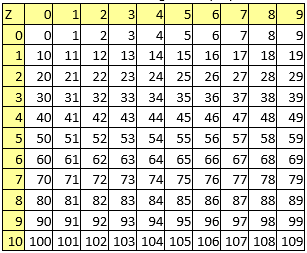In the first element of the list (line 0 column 0 in Table 2) it has been kept the scale of the atomic property - the value to be used to divide all other values when are to be used as atomic property descriptors.

Following tables (from Table 3 to Table 9) lists the constants used for each chemical element.

Table 3. Atomic masses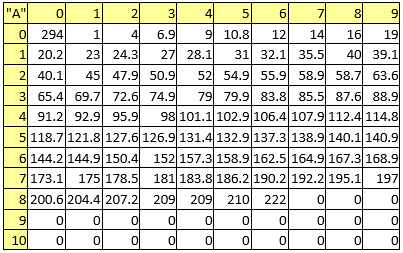Table 4. Boiling points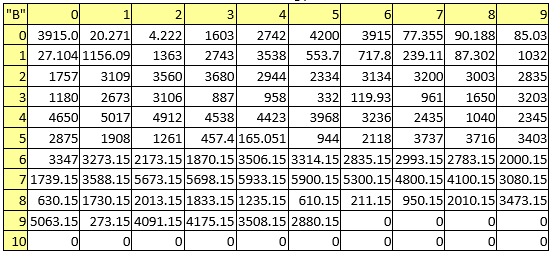Table 5. Densities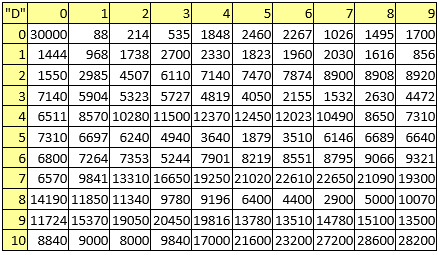Table 6. Electronegativities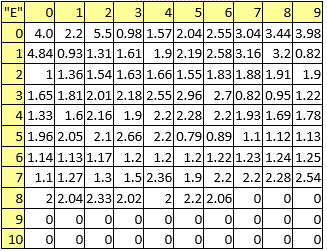Table 7. First ionization potentials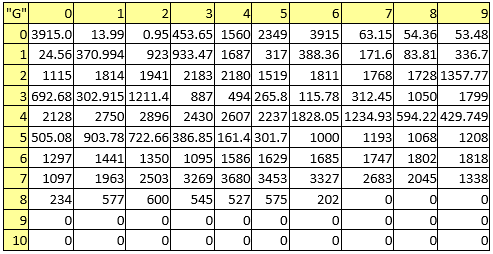Table 8. Melting pointsTable 9. Valences (to be used for Hydrogen connections)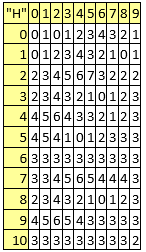The valences (Table 9) are to be used to calculate the number of attached Hydrogen atoms based on the bonds and their orders (by subtracting the sum of the bond orders form the valence), while the counts ("C" property) is trivial - always 1. For the remaining atomic properties ({"I", "J", "K", "L"}), those are molecule-dependent and are expected to be provided from energy calculations on the molecule. As can be observed excepting Hydrogen connections ("H", valences listed in Table 9) the rest of the atomic properties ({"A", "B", "C", "D", "E", "F", "G"}) are molecule-independent.

As exemplified also in Table 1, the connectivities are to be calculated as follows:

• "T" as topological distance matrix ([Di]) provides, inversed ([CT]i,j = 1/[Di]i,j for i ≠ j, 0 otherwise);
• "G" as geometrical distance matrix ([Dxyz]) provides, inversed ([CG]i,j = 1/[Dxyz]i,j for i ≠ j, 0 otherwise);
• "C" and "B" construct first an replacement for adjacency matrix by replacing the values of 1 with the inverse of the bond order ("C" as classical bond orders and "B" as Mulliken bond orders) and calculate a distance matrix ([DC], [DB]) by using this new adjacency matrix, finally inversing those values ([CC]i,j = 1/[DC]i,j for i ≠ j, 0 otherwise; [CB]i,j = 1/[DB]i,j for i ≠ j, 0 otherwise);
• "c" and "b" as dot products of previously calculated [DC] and [DB] matrices with adjacency ([Cc]i,j = [CC]i,j[Ad]i,j; [Cb]i,j = [CB]i,j·[Ad]i,j).

Parameterized by the atomic property (p ∈ {"A", "B", "C", "D", "E", "F", "G", "H" "I", "J", "K", "L"}) and by the connectivity (q ∈ {"t", "g", "c", "b", "T", "G", "C", "B"}) the extended characteristic polynomial become a family of polynomial functions (EChP(λ; p,q)).

3. Practical use of the Characteristic Polynomial extension

It has previously shown in (Bolboacă & Jäntschi) how the classical characteristic polynomial can be used to link the chemical structure with measured properties and activities of the molecules build up from hydrocarbons.

In the same manner the EChP(λ; p, q) may serve to link the chemical structure with measured properties and activities of the molecules build up from any chemical elements.

One strategy is to evaluate the EChP(λ; p, q) polynomial in a series of evenly spaced points from -1 to 1. For three digits rational arguments (λ = -1.000, -0.999, …, -0.001, 0.000, 0.001, …, 0.999, 1.000) are 2001 evaluation points.

By multiplying with the number of alternatives from identity (Ip) and connectivity (Cq) choices (p and q), the number of individuals in the EChP family is 2001·12·8 = 192096. Three linearization operations can be applied ("I", "R", "L") through the functions fI(x) = x, fR(x) = 1/x and fL(x) = ln(x) increasing the number of individuals in the EChP family to 576288. To keep the track of each individual, the naming convention is: L1L2L3C0D1D2D3 where L1 ∈ {"I", "R", "L"} for linearization, L2 ∈ {"A", "B", "C", "D", "E", "F", "G", "H" "I", "J", "K", "L"} for identity, and L3 ∈ {"t", "g", "c", "b", "T", "G", "C", "B"} for connectivity with the correspondences above given, while C is "N" for λ < 0, "0" for λ = 0, and "P" for λ > 0, and D1D2D3 is the group of (3) digits from the evaluating value of λ (varying from "000" to "999").

In (Joița & Jäntschi, 2017) paper is given an application of the use of EChP for a series of C20 fullerene congeners obtained by replacing in a patterned manner carbon atoms with nitrogen and boron. The obtained results revealed good EChP explanatory capabilities of the structure-property relationship for the area (r2adj = 0.994) and for the volume (r2adj = 0.946) of the series of 46 C20 fullerene congeners.

It should be noted that the constants give in Tables 3 to 9 to be used as scaling factors for the atomic properties (as opposite to the atomic properties itself, which were taken from measured or published data) are more or less arbitrary and can be subject to debate, but the principle of constructing EChP is sound. Further studies are necessary to construct a best fit for the scaling factors (eventually made by parameterizing them).

References

1. Euler, L.. De integratione aequationum differentialium altiorum gradurn; Miscellanea Berolinensia 7 = Opera omnia (1) 22 (1936): Basel, 1743; pp. 108-213.
2. D’Alembert, J.. Suite des recherches sur le calcul intégrale; Histoire de l'acadénie des sciences (1750): Berlin, 1748; pp. 249-291.
3. Lagrange, J.-L.. Sur L’équation Séculaire de la Lune; Mémoires de l’Acadéémie Royale des Science (1774): Paris, 1773; pp. 335-399.
4. Laplace, P. S.. Mémoire sur les solutions particulières des équations différentielles et sur les inégalités séculaires des planètes; Mémoires de l’Acadéémie Royale des Science (1775): Paris, 1775; pp. 325-366.
5. Fourier, J. B. J.. Théorie analytique de la chaleur; Chez Firmin Didot & Perè et fils: Paris, 1822; pp. 684.
6. Cauchy, A.-L.. Sur l’équation à l’aide de laquelle on détermine les inégalités séculaires des mouvements des planètes. Exercices de mathématiques.; Rpt. in Oeuvres complètes, série 2, tome 9, 4: Paris, 1829; pp. 174-195.
7. Sylvester, J.J.; Sur une propriété nouvelle de l’équation qui sert à déterminer les inégalités séculaires des planètes. Nouvelles annales de mathématiques, journal des candidats aux écoles polytechnique et normale 1852, 1(11), 434-440, N.A..
8. Hermite, C.; Extrait d'une lettre à M. Borchardt sur l'invariabilité du nombre des carrés positifs et des carrés négatifs dans la transformation des polynômes homogènes du second degré. Journal für die reine und angewandte Mathematik 1857, 53, 271-274, N.A..
9. Weierstrass, K.. Zur theorie der bilinearen und quadratischen formen; Monatsh. Akad. Wiss.: Berlin, 1867; pp. 310-338.
10. Jordan, C.; Sur les oscillations infiniment petites des systèmes matériels. Comptes rendus de l’Académie des sciences de Paris 1872, 74, 1395-1399, N.A..
11. Kronecker, L.. Algebraische reduction der schaaren bilinearer formen; S.-B. Akad.: Berlin, 1890; pp. 763-776.
12. Sylvester, J.J.; On the theorem connected with Newton's rule for the discovery of imaginary roots of equations. Messenger of Mathematics 1880, 9, 71-84, N.A..
13. Schrödinger, E.; An undulatory theory of the mechanics of atoms and molecules. Physical Review 1926, 28(6), 1049-1070, 10.1103/PhysRev.28.1049.
14. D. R. Hartree; The Wave Mechanics of an Atom with a Non-Coulomb Central Field. Part I. Theory and Methods. Mathematical Proceedings of the Cambridge Philosophical Society 1928, 24(1), 89-110, 10.1017/s0305004100011919.
15. D. R. Hartree; The Wave Mechanics of an Atom with a Non-Coulomb Central Field. Part II. Some Results and Discussion. Mathematical Proceedings of the Cambridge Philosophical Society 1928, 24(1), 111-132, 10.1017/s0305004100011920.
16. V. Fock; Näherungsmethode zur Lösung des quantenmechanischen Mehrkörperproblems. The European Physical Journal A 1930, 61(1-2), 126-148, 10.1007/bf01340294.
17. . , , , .
18. J. C. Slater; The Theory of Complex Spectra. Physical Review 1929, 34(10), 1293-1295, 10.1103/PhysRev.34.1293.
19. D. R. Hartree; W. Hartree; Self-consistent field, with exchange, for beryllium. Proceedings of the Royal Society of London. Series A. Mathematical and Physical Sciences 1935, 150(869), 9-33, 10.1098/rspa.1935.0085.
20. Erich Hückel; Quantentheoretische Beiträge zum Benzolproblem. The European Physical Journal A 1931, 70, 204-286, 10.1007/bf01339530.
21. C. A. Coulson; The evaluation of certain integrals occurring in studies of molecular structure. Mathematical Proceedings of the Cambridge Philosophical Society 1937, 33(1), 104-110, 10.1017/s0305004100016820.
22. C. A. Coulson; On the calculation of the energy in unsaturated hydrocarbon molecules. Mathematical Proceedings of the Cambridge Philosophical Society 1940, 36(2), 201-203, 10.1017/s0305004100017175.
23. C. A. Coulson; Notes on the secular determinant in molecular orbital theory. Mathematical Proceedings of the Cambridge Philosophical Society 1950, 46(1), 202-205, 10.1017/s0305004100025639.
24. Dan-Marian Joiţa; Lorentz Jäntschi; Extending the Characteristic Polynomial for Characterization of C20 Fullerene Congeners. Mathematics 2017, 5(4), 84(12), 10.3390/math5040084.
25. Sorana Daniela Bolboaca; Lorentz Jantschi; How Good Can the Characteristic Polynomial Be for Correlations?. International Journal of Molecular Sciences 2007, 8(4), 335-345, 10.3390/i8040335.
More